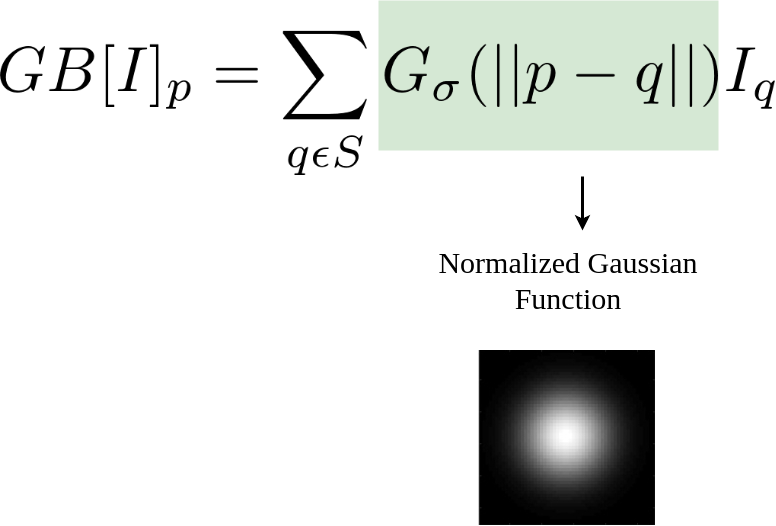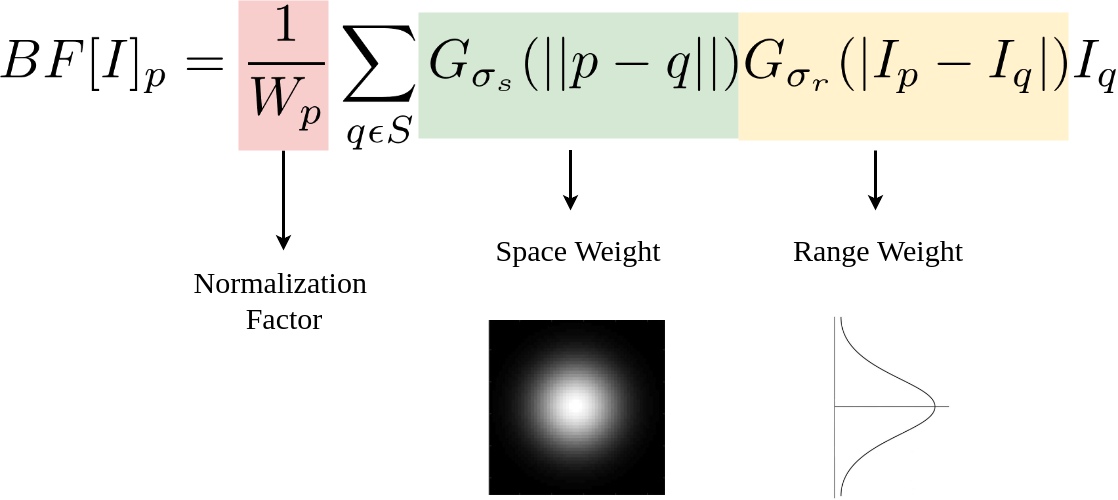Open in App
Not now

# Python | Bilateral Filtering

• Difficulty Level : Hard
• Last Updated : 04 Jan, 2023

A bilateral filter is used for smoothening images and reducing noise, while preserving edges. This article explains an approach using the averaging filter, while this article provides one using a median filter. However, these convolutions often result in a loss of important edge information, since they blur out everything, irrespective of it being noise or an edge. To counter this problem, the non-linear bilateral filter was introduced.

## Gaussian Blur

Gaussian blurring can be formulated as follows:Here,is the result at pixel p, and the RHS is essentially a sum over all pixels q weighted by the Gaussian function.is the intensity at pixel q.

## Bilateral Filter: an Additional Edge Term

The bilateral filter can be formulated as follows:Here, the normalization factor and the range weight are new terms added to the previous equation.denotes the spatial extent of the kernel, i.e. the size of the neighborhood, anddenotes the minimum amplitude of an edge. It ensures that only those pixels with intensity values similar to that of the central pixel are considered for blurring, while sharp intensity changes are maintained. The smaller the value of, the sharper the edge. Astends to infinity, the equation tends to a Gaussian blur.
OpenCV has a function called bilateralFilter() with the following arguments:

1. d: Diameter of each pixel neighborhood.
2. sigmaColor: Value ofin the color space. The greater the value, the colors farther to each other will start to get mixed.
3. sigmaSpace: Value ofin the coordinate space. The greater its value, the more further pixels will mix together, given that their colors lie within the sigmaColor range.

Code :
Input : Noisy Image.Code : Implementing Bilateral Filtering

## Python

 import cv2     # Read the image.  img = cv2.imread('taj.jpg')     # Apply bilateral filter with d = 15,   # sigmaColor = sigmaSpace = 75.  bilateral = cv2.bilateralFilter(img, 15, 75, 75)     # Save the output.  cv2.imwrite('taj_bilateral.jpg', bilateral)

Output of Bilateral FilterComparison with Average and Median filters
Below is the output of the average filter (cv2.blur(img, (5, 5))).Below is the output of the median filter (cv2.medianBlur(img, 5)).Below is the output of the Gaussian filter (cv2.GaussianBlur(img, (5, 5), 0)).It is easy to note that all these denoising filters smudge the edges, while Bilateral Filtering retains them.

My Personal Notes arrow_drop_up
Related Articles